MEMO 2008 pojedinačno problem 3

Kvaliteta:
Avg: 3,0
Težina:
Avg: 5,0
Let$ABC$ be an isosceles triangle with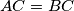$AC = BC$. Its incircle touches$AB$ in$D$ and$BC$ in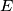$E$. A line distinct of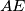$AE$ goes through$A$ and intersects the incircle in$F$ and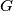$G$. Line$AB$ intersects line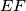$EF$ and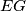$EG$ in$K$ and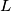$L$, respectively. Prove that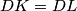$DK = DL$.
Izvor: Srednjoeuropska matematička olimpijada 2008, pojedinačno natjecanje, problem 3